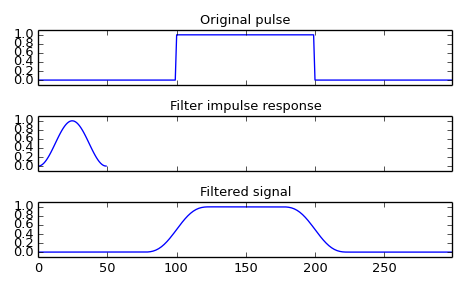# scipy.signal.convolve¶

scipy.signal.convolve(in1, in2, mode='full')[source]

Convolve two N-dimensional arrays.

Convolve in1 and in2, with the output size determined by the mode argument.

Parameters: in1 : array_like First input. in2 : array_like Second input. Should have the same number of dimensions as in1; if sizes of in1 and in2 are not equal then in1 has to be the larger array. mode : str {‘full’, ‘valid’, ‘same’}, optional A string indicating the size of the output: full The output is the full discrete linear convolution of the inputs. (Default) valid The output consists only of those elements that do not rely on the zero-padding. same The output is the same size as in1, centered with respect to the ‘full’ output. convolve : array An N-dimensional array containing a subset of the discrete linear convolution of in1 with in2.

numpy.polymul
performs polynomial multiplication (same operation, but also accepts poly1d objects)

Examples

Smooth a square pulse using a Hann window:

>>> from scipy import signal
>>> sig = np.repeat([0., 1., 0.], 100)
>>> win = signal.hann(50)
>>> filtered = signal.convolve(sig, win, mode='same') / sum(win)

>>> import matplotlib.pyplot as plt
>>> fig, (ax_orig, ax_win, ax_filt) = plt.subplots(3, 1, sharex=True)
>>> ax_orig.plot(sig)
>>> ax_orig.set_title('Original pulse')
>>> ax_orig.margins(0, 0.1)
>>> ax_win.plot(win)
>>> ax_win.set_title('Filter impulse response')
>>> ax_win.margins(0, 0.1)
>>> ax_filt.plot(filtered)
>>> ax_filt.set_title('Filtered signal')
>>> ax_filt.margins(0, 0.1)
>>> fig.tight_layout()
>>> fig.show()#### Previous topic

scipy.signal.vectorstrength

#### Next topic

scipy.signal.correlate